# Linear Queues in Data Structures

Blog Author

Tekslate

Published Date

6th April, 2021

Ratings

Views

0

## Linear Queues

A queue is an ordered list in which items may be added only at one end called the “rear” and items may be removed only at the other end called “front”.

### Examples

1. A line of passengers waiting to buy tickets at a reservation counter. Each new passenger gets in line at the “rear”; the passenger at the front of the lines is reserved.
2. A linear queue can be found in a time-sharing computer system where many users share the system simultaneously.
3. The first element, which is added to the queue will be the first one to be removed. Thus queues are also called First-in First-Out lists (FIFO) or Last-In-Last-Out lists (LILO).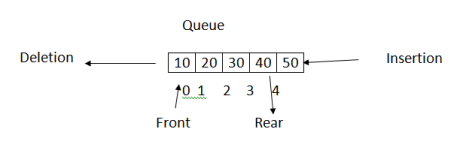• Queues may be represented in the computer memory by means of linear arrays or linked list.
• There are two pointer variables namely FRONT and REAR.
• The FRONT denotes the location of the first element of the queue.
• The rear describes the location of the rear element of the queue.
• When the queue is empty, the values of front and rear are-1 and -1 respectively.

• The “max-size “represents the maximum capacity of the linear queue.
• The following is the condition to test the queue is full or not. (Rear== max size-1)
• To insert the first element both pointers should be altered to ‘0’ Front =0 rear=0.
• Whenever an item is added to the queue, the value of REAR is incremented by 1. REAR =REAR+1;
• The following is the condition to test the queue is empty or not. Front==-1
• Whenever an “Item” is deleted from the queue, the value if the front is incremented by 1 Front=Front+1;
• To delete the last element, both pointers should be altered.

Data Structures Interview Questions

Front=-1; Rear=-1;

Program: Implementation of linear queues using arrays.

``````# include <studio.h>
#include <conio.h>
#include <stdlib.h>
#define maxsize 5
intqueue [maxsize];
int Front=-1;
int Rear=-1;
void Insertion ()
{
intele;
if(Rear== maxsize-1)
{
printf(“Queue is overflow n”);
}
else
{
printf(“Enter an element:”);
sacnf(“%d”,&ele);
Rear=Rear+1;
Queue[Rear]=ele;
if(Front==-1)
{
Front=0;
}
}
}
void deletion ()
{
if(Front==-1)
{
printf(“Queue is under flown”);
}
else
{
printf(“ Deleted element is: %d n”, Queue [Front]);
if (Front==Rear)
{
Front=-1;
Rear=-1;
}
else
{
Front=Front+1;
}
}
}
void display ()
{
inti;
if (Front ==-1)
{
printf(“Queue is under flow n”);
}
else
{
for(i= Front; i<= Rear; i++)
{
prinft(“%5d”,Queue[i]);
}
printf(“n”);
}
}
void main()
{
int choice;
clrscr();
int choice;
printf(“1.INSERTION n”);
printf(“2.DELETION  n”);
printf(“3.DISPLAY  n”);
printf(“4.EXIT n”);
do
{
scanf(“%d”,&choice);
switch (choice)
{
case1: insertion (); break;
case2: deletion (); break;
case3: Display(); break;
case4: (0);
}
}
while (choice <=4)
}``````

1). Initial State: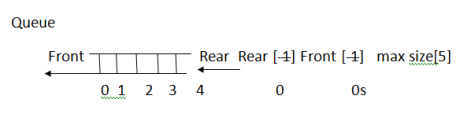2). After inserting element 10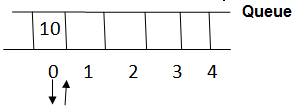3). After inserting elements 20, 30, 40, 50: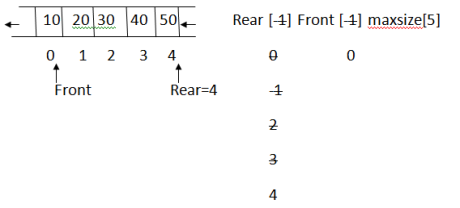4). Inserting element 60 Queue is over flow   5).After deleting elements 10,20,30,40: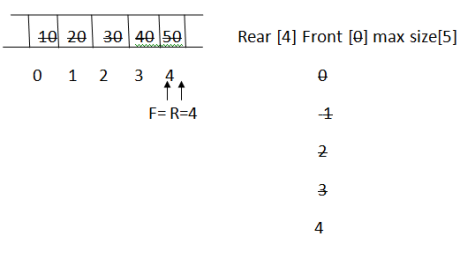5). After inserting elements 20, 30, 40, 50: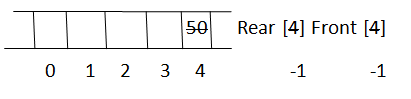## Applications of the queue:

• A queue can be used to store a lift of interrupts t=in the operating system, which would get processed in the order in which they were generated.
• A queue can be found in a time-sharing computer system where many users share the system simultaneously.
• In a computer network, messages from one to the computer generally create asynchronously. These messages, therefore, need to be buffered until the receiving computer is ready for id. These communication buffers make extensive use of queues by staring these messages in a queue. Also, the messages need to be sent to receiving computer in the same order in which they are created, i.e. FIFO order.